# Casorati-Sokhotskii-Weierstrass theorem

(diff) ← Older revision | Latest revision (diff) | Newer revision → (diff)

Weierstrass theorem, Weierstrass–Sokhotskii–Casorati theorem

Letbe an essential singular point of an analytic functionof a complex variable. Given any complex number(including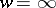), there is a sequence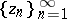converging tosuch that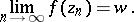This theorem was the first result characterizing the cluster set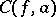of an analytic functionat an essential singularity. According to the theorem,is total, that is, it coincides with the extended plane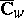of the variable. The theorem was proved by Yu.V. Sokhotskii  (see also ). K. Weierstrass stated this theorem in 1876 (see ). Further information about the behaviour of an analytic function in a neighbourhood of an essential singularity is contained in the Picard theorem.

This theorem does not admit a direct generalization to the case of analytic mappingsfor(see ).

How to Cite This Entry:
Casorati-Sokhotskii-Weierstrass theorem. Encyclopedia of Mathematics. URL: http://encyclopediaofmath.org/index.php?title=Casorati-Sokhotskii-Weierstrass_theorem&oldid=11218
This article was adapted from an original article by E.D. Solomentsev (originator), which appeared in Encyclopedia of Mathematics - ISBN 1402006098. See original article